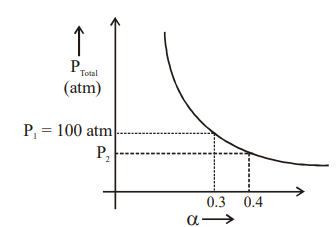# For following equilibrium:  ; ${\mathrm{K}}_{\mathrm{P}}=\frac{{\mathrm{P}}_{{\mathrm{NO}}_{2}}^{2}}{{\mathrm{P}}_{{\mathrm{N}}_{2}{\mathrm{O}}_{4}}}$; Graph between  $\alpha$ is given. Where ${P}_{total}$ = Total pressure at equilibrium a ® degree of dissociation (a << 1)1. A

value of ${K}_{P}$ is

2. B

value of ${P}_{2}$ is

3. C

If total pressure is increased at equilibrium then reaction will move in backward direction.

4. D

On increasing temperature degree of dissociation of  will increase.

FREE Lve Classes, PDFs, Solved Questions, PYQ's, Mock Tests, Practice Tests, and Test Series!

+91

Verify OTP Code (required)

I agree to the terms and conditions and privacy policy.

### Solution:

so if ${P}_{t}$ increases then $\alpha$ decreases to reset ${Q}_{P}={K}_{P}.$

$\left(4\right)$ As reaction is endothermic in forward direction so on increasing temperature, it favour endothermic step i.e. $\alpha$ increases.

## Related content

Join Infinity Learn Regular Class Program!

Sign up & Get instant access to FREE PDF's, solved questions, Previous Year Papers, Quizzes and Puzzles!

+91

Verify OTP Code (required)

I agree to the terms and conditions and privacy policy.